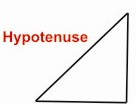HOME MATH DICTIONARY DOWNLOAD FEEDBACK DISCLAIMER
 Question: What is Right Angled Triangle ? Answer: This is a triangle in which one angle is a right angle. The side opposite to the right angle is called the hypotenuse. A right angled triangle has one 90o internal angle (a right angle). The side opposite to the right angle is the hypotenuse, it is the longest side in the right triangle. The other two sides are the legs or catheti of the triangle. Example of a Right Angled Triangle :